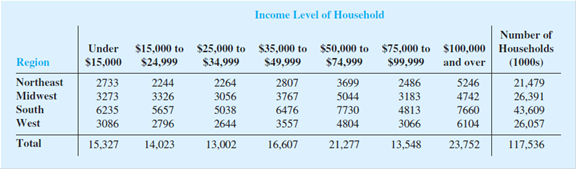# The following crosstabulation shows the number of households (1000s) in each of the four regions of the United States and the number of households at each income level (U.S. Census Bureau website, August 2013). a. Compute the row percentages and identify the percent frequency distributions of income for households in each region. b. What percentage of households in the west region have an income level of $50,000 or more? What percentage of households in the South region have an income level of$50,000 or more? c. Construct percent frequency histograms for each region of households. do any relationships between regions and income level appear to be evident in your findings? d. Compute the column percentages. What information do the column percentages provide? e. What percent of households with a household income of $100,000 and over are from the South region? What percentage of households from the South region have a household income of$100,000 and over? Why are these two percentages different?13th Edition
Anderson
Publisher: CENGAGE L
ISBN: 978130588188413th Edition
Anderson
Publisher: CENGAGE L
ISBN: 9781305881884

#### Solutions

Chapter
Section
Chapter 2.3, Problem 32E
Textbook Problem
500 views

## The following crosstabulation shows the number of households (1000s) in each of the four regions of the United States and the number of households at each income level (U.S. Census Bureau website, August 2013).a. Compute the row percentages and identify the percent frequency distributions of income for households in each region. b. What percentage of households in the west region have an income level of $50,000 or more? What percentage of households in the South region have an income level of$50,000 or more? c. Construct percent frequency histograms for each region of households. do any relationships between regions and income level appear to be evident in your findings? d. Compute the column percentages. What information do the column percentages provide? e. What percent of households with a household income of $100,000 and over are from the South region? What percentage of households from the South region have a household income of$100,000 and over? Why are these two percentages different?

Expert Solution

a.

To determine

Compute the row percentage for the data and identify the percentage frequency distribution of income for household in each region.

### Explanation of Solution

Calculation:

The data represents the cross tabulation for the number of household in each of the four regions of the United States and the number of households income level of household.

Software procedure:

Step-by-step procedure to compute row percent’s is given below:

• Choose stat>tables>cross tabulation and chi square.
• Choose raw data (summarized).
• In rows, enter the column of region.
• In columns, enter the column of as with the limits of income level of household.
• Under display, select row percent.
• Click OK

Output using the MINITAB software for the row percent is given below:

Each row of the above table represents each of the region’s percent frequency distribution.

Therefore, the following table represents the percent frequency for west region...

Expert Solution

b.

To determine

Find the percentage of households in the west region have an income level of $50,000 or more. Find the percentage of households in the South region have an income level of$50,000 or more.

Expert Solution

c.

To determine

Construct the percent frequency histogram for each of the households region.

Check whether any relationship between the region and income level appear to be evident in the output findings.

Expert Solution

d.

To determine

Compute the column percentage for the data

Find the information provided by the column percentage.

Expert Solution

e.

To determine

Find the percent of households with a household income of $100,000 and over from the south region. Find the percentage of households with a households from the south region have a household income of$100,000 and more.

Find the reason for the difference that exists among these two percentages.

### Want to see the full answer?

Check out a sample textbook solution.See solution

### Want to see this answer and more?

Bartleby provides explanations to thousands of textbook problems written by our experts, many with advanced degrees!

See solution

Find more solutions based on key concepts
Show solutions
Why is a call provision advantageous to a bond issuer? When would the issuer be likely to initiate a refunding ...

Fundamentals of Financial Management, Concise Edition (with Thomson ONE - Business School Edition, 1 term (6 months) Printed Access Card) (MindTap Course List)

Describe how to account for organization costs.

College Accounting, Chapters 1-27 (New in Accounting from Heintz and Parry)

Sometimes, Always, or Never: If an ≥ bn ≥ 0 for all n and {bn} diverges, then {an} diverges.

Study Guide for Stewart's Single Variable Calculus: Early Transcendentals, 8th

Perform the indicated operation for the following. 8.

Contemporary Mathematics for Business & Consumers

Calculating with Quotients of Polynomials Exercises 9 through 12 involve limits of quotients of polynomials. Ei...

Functions and Change: A Modeling Approach to College Algebra (MindTap Course List)

Solve the equations in Exercises 112 for x (mentally, if possible). x+1=2x+2

Finite Mathematics and Applied Calculus (MindTap Course List)

In Exercises 4449, find an equation of the line L that passes through the point (2, 4) and satisfies the condit...

Applied Calculus for the Managerial, Life, and Social Sciences: A Brief Approach

Give the metric prefix for each value: 1000

Elementary Technical Mathematics

The area of the shaded region is given by:

Study Guide for Stewart's Multivariable Calculus, 8th

Movie Critic Opinions. On a television program, two movie critics provide their reviews of recent movies and di...

Modern Business Statistics with Microsoft Office Excel (with XLSTAT Education Edition Printed Access Card) (MindTap Course List)

In Problem 35 determine the equation of motion if the external force is f(t) = et sin 4t. Analyze the displacem...

A First Course in Differential Equations with Modeling Applications (MindTap Course List)